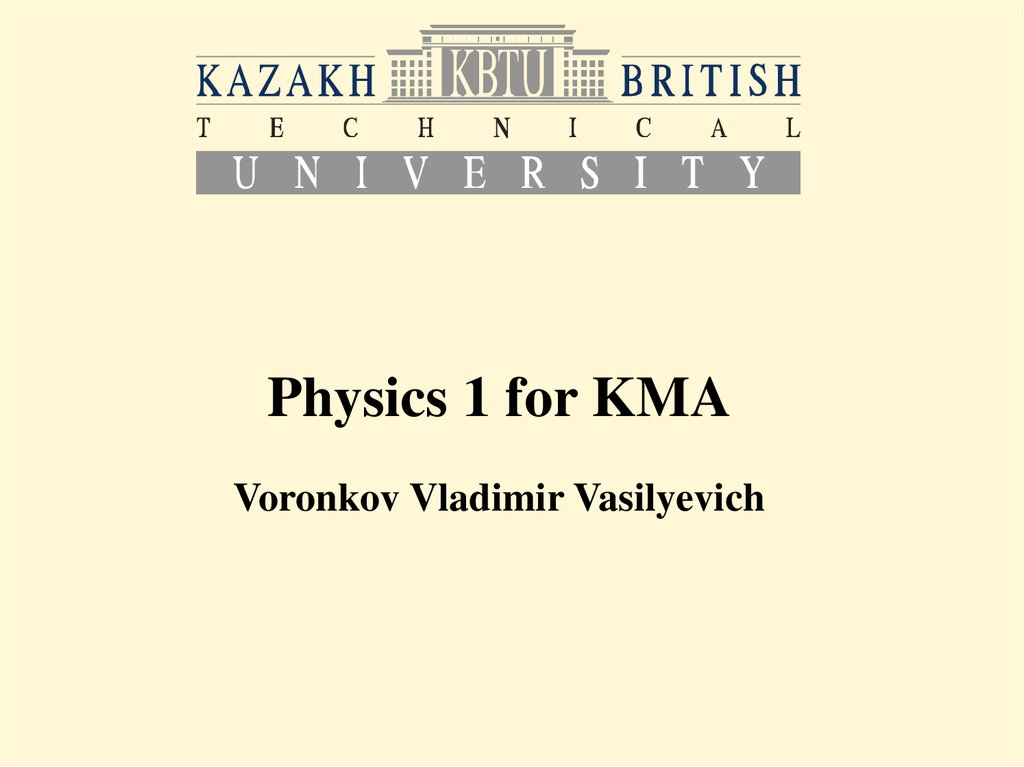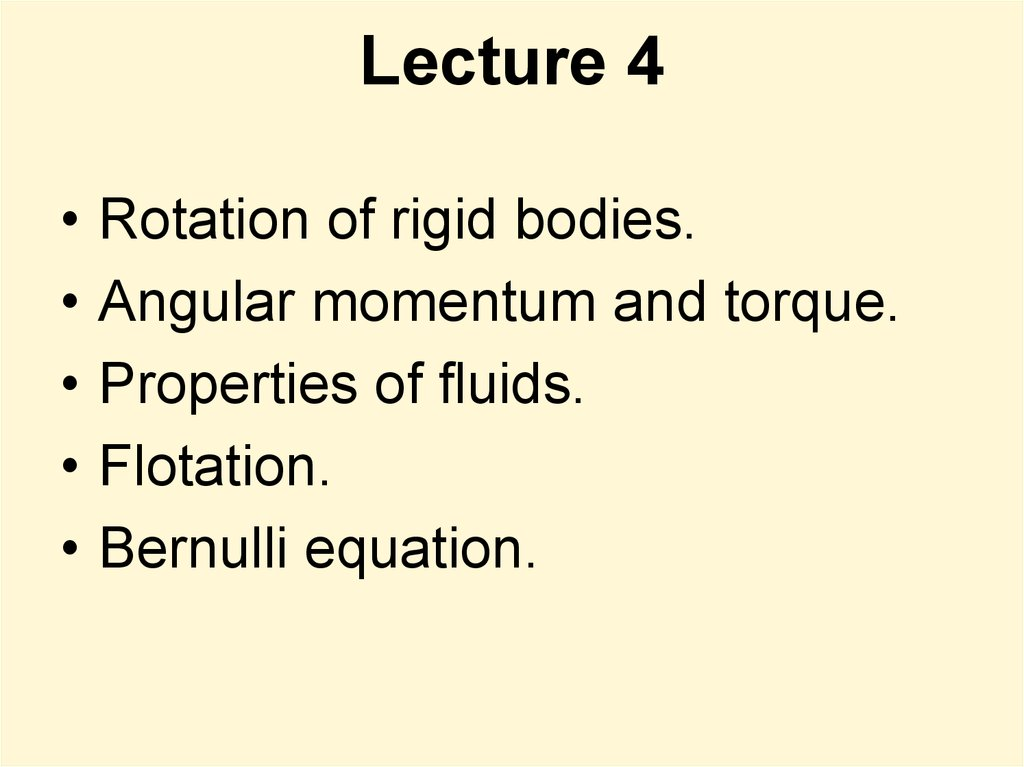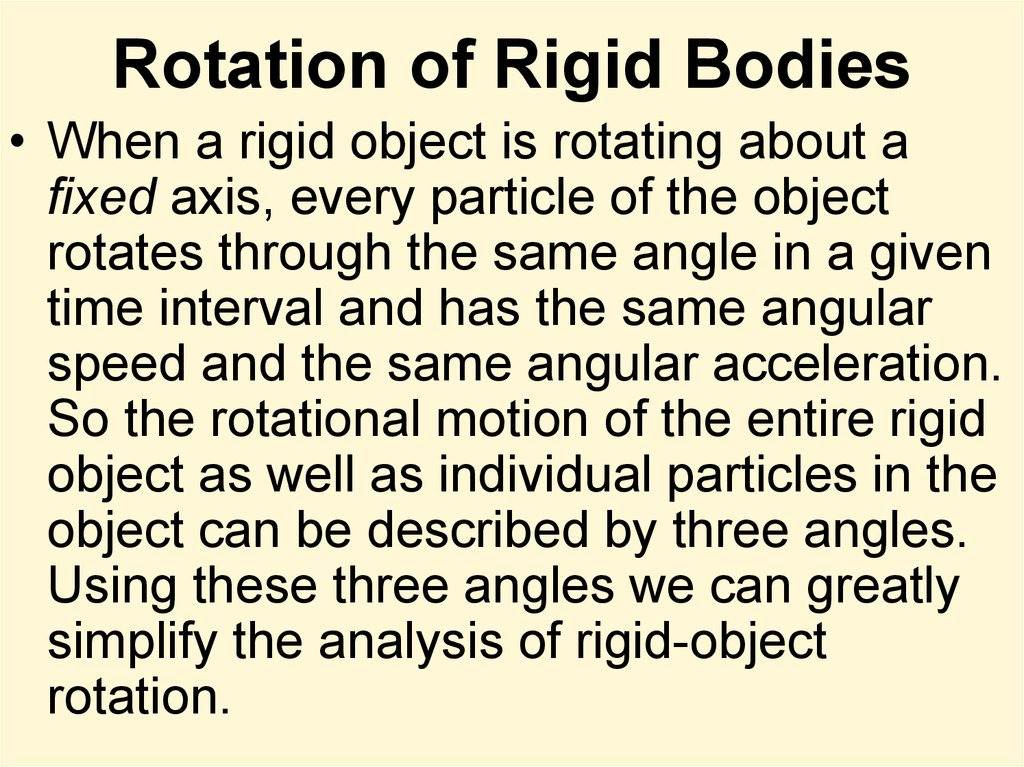# Physics 1 for KMA

## 1.

Physics 1 for KMA

## 2. Lecture 4

Rotation of rigid bodies.
Angular momentum and torque.
Properties of fluids.
Flotation.
Bernulli equation.

## 3. Rotation of Rigid Bodies

• When a rigid object is rotating about a
fixed axis, every particle of the object
rotates through the same angle in a given
time interval and has the same angular
speed and the same angular acceleration.
So the rotational motion of the entire rigid
object as well as individual particles in the
object can be described by three angles.
Using these three angles we can greatly
simplify the analysis of rigid-object
rotation.

ratio of the arc length s and

## 5. Angular kinematics

• Angular displacement:
• Instantaneous angular
speed:
• Instantaneous angular
acceleration:

## 6. Angular and linear quantities

• Every particle of the object moves in a
circle whose center is the axis of rotation.
• Linear velocity:
• Tangential acceleration:
• Centripetal acceleration:

## 7. Total linear acceleration

• Tangential acceleration is perpendicular to
the centripetal one, so the magnitude of
total linear acceleration is

## 8. Angular velocity

• Angular velocity is a vector.
The right hand
rule is applied: If
the fingers of
curl along with
the rotation your
thumb will give
the direction of
the angular
velocity.

## 9. Rotational Kinetic Energy

• Moment of rotational
inertia
• Rotational kinetic
energy

## 16. Parallel-axis theorem

• Suppose the moment of inertia about an
axis through the center of mass of an
object is ICM. Then the moment of inertia
about any axis parallel to and a distance D
away from this axis is

## 18. Torque

When a force is exerted on a rigid object
pivoted about an axis, the object tends to
rotate about that axis. The tendency of a
force to rotate an object about some axis
is measured by a vector quantity called
torque t (Greek tau).

## 19.

The force F has a greater rotating
tendency about axis O as F increases and
as the moment arm d increases. The
component F sinf tends to rotate the

## 20.

The force F1 tends to rotate the
O, and F2 tends to rotate it
clockwise.
We use the convention that the sign of the
torque resulting from a force is positive if the
turning tendency of the force is
counterclockwise and is negative if the
turning tendency is clockwise. Then

## 21. Torque is not Force Torque is not Work

Torque should not be confused with force. Forces can
cause a change in linear motion, as described by
Newton’s second law. Forces can also cause a
change in rotational motion, but the effectiveness of
the forces in causing this change depends on both
the forces and the moment arms of the forces, in the
combination that we call torque. Torque has units of
force times length—newton · meters in SI units—and
should be reported in these units.
Do not confuse torque and work, which have the
same units but are very different concepts.

## 22. Rotational Dynamics

and
Then:
get
which equals zero, as
are parallel.
So we

## 23. Rotational analogue of Newton’s second law

• Quantity L is an instantaneous
angular momentum.
• The torque acting on a particle is
equal to the time rate of change of
the particle’s angular momentum.

## 24. Net External Torque

The net external torque acting on a
through an origin in an inertial frame
equals the time rate of change of the
total angular momentum of the

## 25. Angular Momentum of a Rotating Rigid Object

• Angular momentum for each particle of an
object:
• Angular momentum for the whole object:
• Thus:

## 27. The Law of Angular Momentum Conservation

• The total angular momentum of a
system is constant if the resultant
external torque acting on the system
is zero, that is, if the system is
isolated.

## 28.

• Change in internal structure of a rotating
body can result in change of its angular
velocity.

## 29.

• When a rotating skater pulls his hands
towards his body he spins faster.

Full mechanical
energy, linear
momentum and
angular
momentum of an
isolated system
remain constant.

## 31. Work-Kinetic Theory for Rotations

• Similarly to linear motion:

## 32.

• The net work done by external
forces in rotating a symmetric
rigid object about a fixed axis
equals the change in the object’s
rotational energy.

## 34. Gyroscope

One typical type of
by suspending a
relatively massive
rotor inside three
rings called
gimbals. Mounting
each of these rotors
on high quality
bearing surfaces
insures that very
little torque can be
exerted on the
inside rotor.

## 35.

At high speeds, the gyroscope exhibits
extraordinary stability of balance and maintains
the direction of the high speed rotation axis of its
central rotor. The implication of the conservation
of angular momentum is that the angular
momentum of the rotor maintains not only its
magnitude, but also its direction in space in the
absence of external torque. The classic type
gyroscope finds application in gyro-compasses.

## 36.

If a gyroscope is tipped,
the gimbals will try to
reorient to keep the spin
axis of the rotor in the
same direction. If
released in this
orientation, the
gyroscope will precess
in the direction shown
because of the torque
exerted by gravity on the
gyroscope.

## 38. Fluids and liquids

• Fluids = liquids + gases
• Fluids flow. They include liquids and gases.
Liquids are a type of fluid that flows and takes
the shape of its container but does not expand to
fill its container. (Gases do that.) Liquid is the
second state of matter, between solid and gas.
• Liquids do not expand, gases do. Gases and
liquids are both fluids.

## 39. Relative density

• Relative density or specific gravity is the ratio of
the density of a substance to the density of a given
reference material. Specific gravity usually means
relative density with respect to water.
• If the reference material is water then a substance
with a relative density (or specific gravity) less than 1
will float in water. For example, an ice cube, with a
relative density of about 0.91, will float. A substance
with a relative density greater than 1 will sink.

Substance
Relative
density
Substance
Relative
density
Alcohol
0.82
Nylon
1.12
Mercury
13.95
PVC
1.36
Paraffin
0.80
Rubber
0.96
Petrol
0.72
Steel
7.82
Water (4oC)
1.00
Tin
7.28
Sea water
1.02
Zinc
7.12
Aluminum
2.72
Acetylene
0.0017
Brass
8.48
8.57
Dry air
0.0013
Chromium
7.03
Carbon dioxide
0.00198
Copper
8.79
Carbon monoxide
0.00126
Cast iron
7.20
Hydrogen
0.00009
11.35
Nitrogen
0.00125
Nickel
8.73
Oxygen
0.00143

## 41.

• Specific volume of a substance is the ratio
of the substance's volume to its mass. It is
the reciprocal of density and is an intrinsic
property of matter:

## 43. Manometer

• The difference in fluid height in
a liquid column manometer is
proportional to the pressure
difference.
• P1-P2=rgh

## 44. Static Fluid Pressure

Pstatic fluid = ρgh
where
ρ = m/V = fluid density
g = gravitational acceleration
h = depth of fluid
• The pressure exerted by a static fluid depends only upon the
depth of the fluid, the density of the fluid, and the acceleration
of gravity

## 45. Pressure Thrust

• Thrust is a total force in a particular
direction. The unit of thrust, therefore is
the same as that of force: Newtons (N).
Pressure is the force or thrust applied per
unit area.
F=P·A

## 46. Atmospheric Pressure

• The surface of the earth is at the bottom of an
atmospheric sea. The standard atmospheric
pressure is measured in various units:
• 1 atmosphere = 760 mmHg = 101.3 KPa
• The bar is a unit of pressure defined as 100
kilopascals. It is about equal to the atmospheric
pressure on Earth at sea level.
• The unit mmHg is often called torr, particularly
in vacuum applications: 760 mmHg = 760 torr

## 49. Aneroid barometer

• An aneroid barometeru ses a small, flexible
metal box called an aneroid cell (capsule),
which is made from an alloy of beryllium and
copper. The evacuated capsule (or usually
more capsules) is prevented from collapsing by
a strong spring. Small changes in external air
pressure cause the cell to expand or contract.
This expansion and contraction drives
mechanical levers such that the tiny
movements of the capsule are amplified and
displayed on the face of the aneroid barometer.

## 51. The Barometric Formula

mair=28.9644 g/mol
mair= mair/Na

## 52. Pascal's Principle

• Pressure exerted anywhere in a
confined incompressible fluid is
transmitted equally in all directions
throughout the fluid such that the
pressure ratio (initial difference)
remains the same.

## 55. Lift pump

The lift pump, also known as a suction
pump, operates as follows:
- on the upstroke of the plunger, the lower
valve opens, the upper valve (situated on or
in the plunger itself) is closed, and the low air
pressure produced in the barrel allows
atmospheric pressure on the surface of the
water source, down below, to make the water
move up the downpipe and eventually fill the
barrel below the plunger.
- On the downstroke, the lower valve closes,
the upper one opens, and water is forced
into the barrel above the upper valve. On the
next upstroke, the water above the plunger is
forced out of the spout, located at the top of
the barrel, at the same time as the volume
below the barrel fills up with water again.

## 56. Force pump

The force pump, also known as a
pressure pump, operates as
follows:
- on the upstroke of the plunger, the
outlet or delivery valve is closed and
the inlet valve opens. The low air
pressure produced in the barrel
causes the water below to move up
the downpipe and eventually fill the
barrel.
- On the downstroke, the inlet valve
closes, the outlet valve opens, and
the water is forced out via the outlet
pipe, which is located at the bottom
of the barrel.

Rotary vane pump
Scroll pump

## 58. Height limitation

Total Dynamic Head (TDH) is the total
equivalent height that a fluid is to be
pumped, taking into account friction losses
in the pipe.

## 59. Total Dynamic Height

TDH = Static Height + Static Lift + Friction Loss
Static Height is the maximum height reached by the pipe
after the pump (also known as the 'discharge head').
Static Lift is the height the water will rise before arriving
at the pump (also known as the suction head).
Friction Loss - in any real moving fluid, energy is
dissipated due to friction; turbulence dissipates even
more energy for high Reynolds number flows. Friction
loss is divided into two main categories, "major losses"
associated with energy loss per length of pipe, and "minor
losses" associated with bends, fittings, valves, etc.

## 60. Viscosity

The resistance to flow of a fluid and the resistance
to the movement of an object through a fluid are
usually stated in terms of the viscosity of the fluid.
dv
F A
dy

## 61.

Experimentally, under conditions of laminar flow,
the force required to move a plate at constant
speed against the resistance of a fluid is
proportional to the area of the plate and to the
velocity gradient perpendicular to the plate. The
constant of proportionality is called the viscosity .

## 63. Drag force due viscosity

In a viscous fluid, a boundary layer is formed. This
causes a net drag due to skin friction. Further,
because the ideal pressure now acts on the boundary
layer, as opposed to the ship, and the boundary layer
grows along the length of the ship, the net opposing
forces are greater than the net supporting forces.
This further adds to the resistance.

## 64. Effect of Temperature on Viscosity

The temperature dependence of liquid viscosity is the
phenomenon by which liquid viscosity tends to
decrease (or, alternatively, its fluidity tends to
increase) as its temperature increases.
0 exp b T
here 0 and b are constants.
This is an empirical model that usually works for a
limited range of temperatures.

## 65. Liquid Damping

• Damping is an effect that reduces the amplitude
of oscillations in an oscillatory system
• Fluid viscous damping is a way to add energy
dissipation to the lateral system of a building
structure. A fluid viscous damper dissipates
energy by pushing fluid through an orifice,
producing a damping pressure which creates a
force. These damping forces are 90 degrees out
of phase with the displacement driven forces in
the structure. This means that the damping force
does not significantly increase the seismic loads
for a comparable degree of structural deformation.

## 68. Buoyancy

Buoyancy arises from the fact that fluid pressure
increases with depth and from the fact that the
increased pressure is exerted in all directions
(Pascal's principle) so that there is an unbalanced
upward force on the bottom of a submerged object.

## 69. Archimedes' Principle

• The buoyant force on a submerged
object is equal to the weight of the
fluid displaced.
• The upward thrust which the surrounding
fluid exerts on an object is referred to as
the force of buoyancy.

## 70. Hydrometer

A hydrometer is an instrument used to measure the
specific gravity (or relative density) of liquids;
that is, the ratio of the density of the liquid to the
density of water.
• A hydrometer is usually made of glass and consists
of a cylindrical stem and a bulb weighted with
mercury or lead shot to make it float upright. The
liquid to be tested is poured into a tall container,
often a graduated cylinder, and the hydrometer is
gently lowered into the liquid until it floats freely.
The point at which the surface of the liquid touches
the stem of the hydrometer is noted. Hydrometers
usually contain a scale inside the stem, so that the
specific gravity can be read directly. A variety of
scales exist, and are used depending on the
context.
• Hydrometers may be calibrated for different uses,
such as a lactometer for measuring the density
(creaminess) of milk, a saccharometer for
measuring the density of sugar in a liquid, or an
alcoholometer for measuring higher levels of
alcohol in spirits.

## 72.

Determine, what liquid is
denser?

## 73.

This liquid is denser.
This liquid is lighter.

## 74. Fluid Kinetic Energy

• The kinetic energy of a moving fluid is
more useful in applications like the
Bernoulli equation when it is expressed as
kinetic energy per unit volume

## 75. Fluid Potential Energy

• The potential energy of a moving fluid is
more useful in applications like the
Bernoulli equation when is expressed as
potential energy per unit volume

## 77. Venturi meter

The Venturi effect is the reduction in fluid pressure
that results when a fluid flows through a
constricted section of pipe.
The Venturi effect is named after Giovanni Battista
Venturi (1746–1822), an Italian physicist.

## 78. Venturi effect

Q is volumetric flow rate
So Venturi meter can be used to measure the flow rate.

## 79. Water Eductor

• Liquid Jet Eductors use the kinetic energy of a
motive liquid to entrain another liquid,
completely mix the two, and then discharge the
mixture against a counter pressure and are
used for pumping and mixing operations.